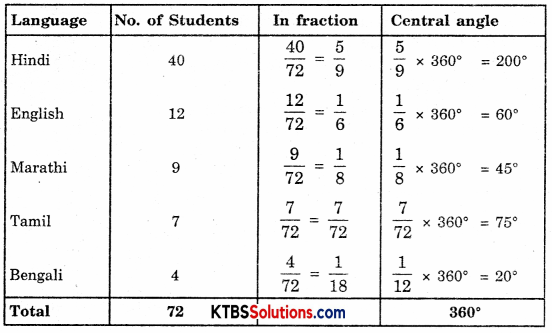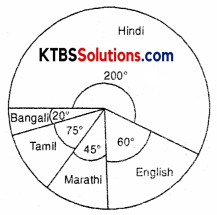# KSEEB Solutions for Class 8 Maths Chapter 5 Data Handling Ex 5.2

## KSEEB Solutions for Class 8 Maths Chapter 5 Data Handling Ex 5.2

Question 1.
A survey was made to find the type of music that a certain group of young people liked in a city. The adjoining pie chart shows the findings of this survey.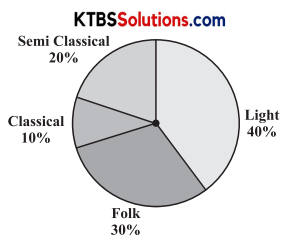From this pie chart answer the following:
(i) If 20 people liked classical music, how many young people were surveyed?
(ii) Which type of music is liked by the maximum number of people?
(iii) If a cassette company were to make 1000 CD’s how many of each type would they make?
Solution:
(i) Number of people who liked classical music = 20
If 10% people liked classical music the total people = 20
1% people liked classical music = $$\frac{20}{10 \%}$$
100% people liked classical music = $$\frac{20}{10} \times 100$$ = 200 People

(ii) Light music

(iii) Total CD’s = 1000
Semi-classical CD’s = 20%
∴ Number of Semi-classical CD’s = 20% of 1000
= $$\frac{20}{100} \times 1000$$
= 200
Number of Classical CD’s = 10% of 1000
= $$\frac{10}{100} \times 1000$$
= 100
Number of Folk CD’s = 30% of 1000
= $$\frac{30}{100} \times 1000$$
= 300
Number of Light music CD’s = 40% of 1000
= $$\frac{40}{100} \times 1000$$
= 400Question 2.
A group of 360 people was asked to vote for their favourite season from the three seasons rainy, winter, and summer.
(i) Which season got the most votes?
(ii) Find the central angle of each sector.
(iii) Draw a pie chart to show this information.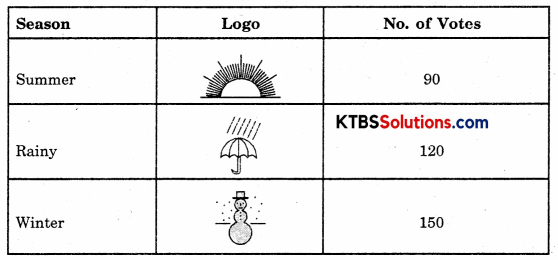Solution:
(i) Winter
(ii) Central Angle = $$\frac{\text { Number of votes }}{\text { Total votes }} \times 360^{\circ}$$
∴ Central angle for Summer = $$\frac{90}{360} \times 360^{\circ}$$ = 90°
Central angle for Rainy Season = $$\frac{120}{360} \times 360^{\circ}$$ = 120°
Central angle for Winter Season = $$\frac{150}{360} \times 360^{\circ}$$ = 150°
(iii)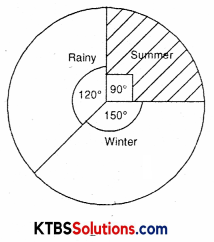Question 3.
Draw a pie chart showing the following information, the table shows the colours preferred by a group of people.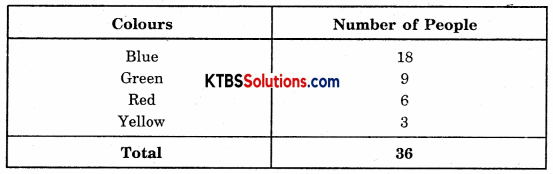Find the proportion of each sector. For example,
Blue is = $$\frac{18}{36}=\frac{1}{2}$$; Green is = $$\frac{9}{36}=\frac{1}{4}$$ and so on. Use this to find the corresponding angles.
Solution: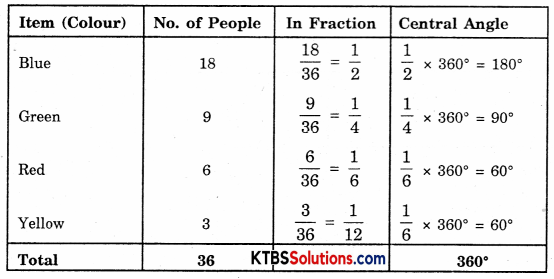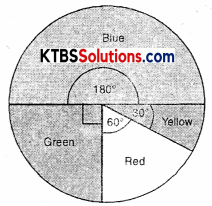Question 4.
The adjoining pie chart gives the marks scored in an examination by a student in Hindi, English, Mathematics, Social Science, and Science. If the total marks obtained by the students were 540, answer the following questions: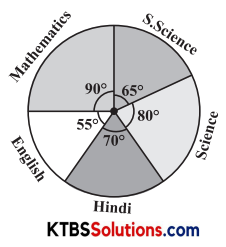(i) In which subject did the student score 105 marks?
(Hint: for 540 marks, the central angle = 360°. So, for 105 marks, what is the central angle?)
(ii) How many more marks were obtained by the student in Mathematics than in Hindi?
(iii) Examine whether the sum of the marks obtained in Social Science and Mathematics is more than that in Science and Hindi.
(Hint: Just study the central angles).
Solution:
(i) For 540 marks, Central angle = 360°
For 1 mark, Central angle = $$\frac{360^{\circ}}{540}$$
For 105 marks, Central angle = $$\frac{360^{\circ}}{540} \times 105$$ = 70°
It shows that if a student score 105 marks, it is represented on pie chart with 70° angle. Hence, the subjects is Hindi.(ii) For 360° marks obtained = 540
For 1° marks obtained = $$\frac{540}{360^{\circ}}$$
For 90° marks obtained = $$\frac{540}{360^{\circ}} \times 90^{\circ}$$ = 135
In Maths mark obtained = 135
Difference of marks in Maths and Hindi = 135 – 105 = 30

(iii) Marks obtained in Social Science = $$\frac{540}{360^{\circ}} \times 65^{\circ}$$ = 97.5
Marks obtained in Science = $$\frac{540}{360^{\circ}} \times 80^{\circ}$$ = 120°
Total marks obtained in (Social Science + Math) = 97.5 + 135 = 232.5
Total marks obtained in (Science + Hindi) = 80 + 70 = 150

Question 5.
The number of students in a hostel, speaking different languages is given below. Display the data, in a pie chart.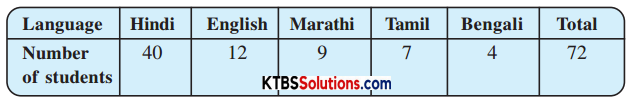Solution: